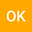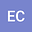The Solution of The Linear Delay Differential Equations with Aboodh Transform
••Özgür KOTAN
Ataturk University

Corresponding Author:ozgurkotan2525@gmail.com

Author Profile## Abstract

In this paper, we apply Aboodh transform to solve linear delay differential equations. Firstly, The basic properties of the Aboodh Transform are given. Secondly, Existence of the Aboodh transform proved. Then, the two linear delay differential equations are solved by Aboodh transform. This means that Aboodh transform is a powerful tool for solving linear delay differential equations.